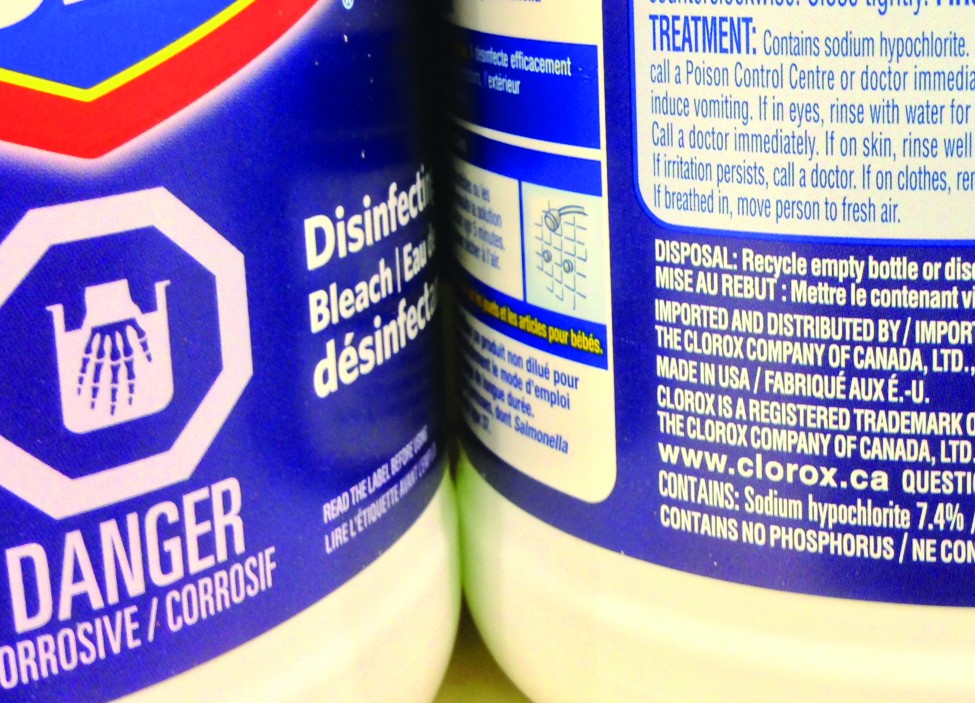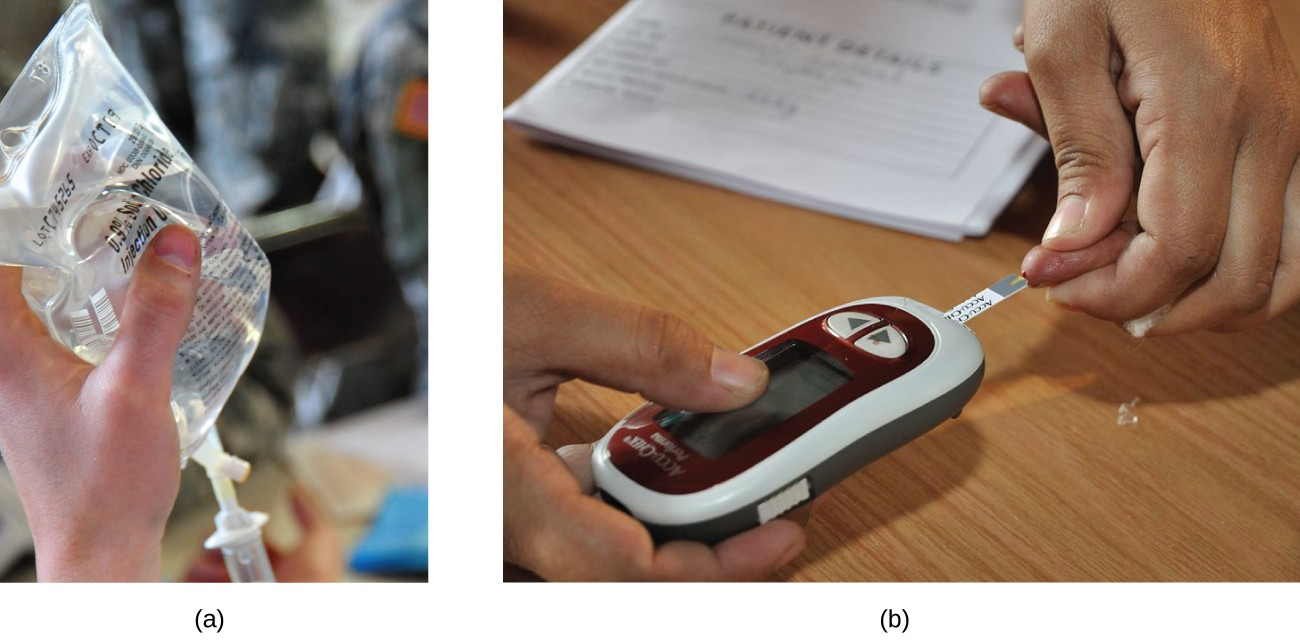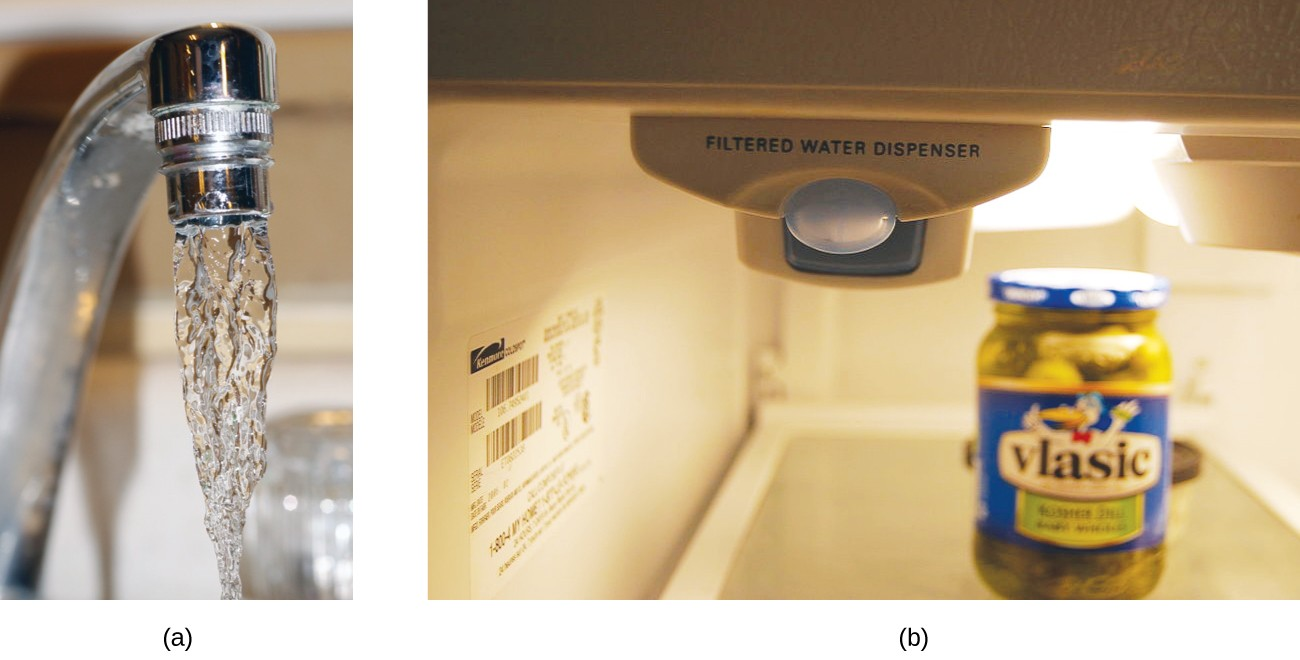## Other Units for Solution Concentrations

### Learning Outcomes

• Define the concentration units of mass percentage, volume percentage, mass-volume percentage, parts-per-million (ppm), and parts-per-billion (ppb)
• Perform computations relating a solution’s concentration and its components’ volumes and/or masses using these units

In the previous section, we introduced molarity, a very useful measurement unit for evaluating the concentration of solutions. However, molarity is only one measure of concentration. In this section, we will introduce some other units of concentration that are commonly used in various applications, either for convenience or by convention.

## Mass Percentage

Earlier, we learned about percent composition as a measure of the relative amount of a given element in a compound. Percentages are also commonly used to express the composition of mixtures, including solutions. The mass percentage of a solution component is defined as the ratio of the component’s mass to the solution’s mass, expressed as a percentage:

$\text{mass percentage}=\dfrac{\text{mass of component}}{\text{mass of solution}}\times 100\%$

We are generally most interested in the mass percentages of solutes, but it is also possible to compute the mass percentage of solvent.

Mass percentage is also referred to by similar names such as percent mass, percent weight, weight/weight percent, and other variations on this theme. The most common symbol for mass percentage is simply the percent sign, %, although more detailed symbols are often used including %mass, %weight, and (w/w)%. Use of these more detailed symbols can prevent confusion of mass percentages with other types of percentages, such as volume percentages (to be discussed later in this section).

Mass percentages are popular concentration units for consumer products. The label of a typical liquid bleach bottle (Figure 1) cites the concentration of its active ingredient, sodium hypochlorite (NaOCl), as being 7.4%. A 100.0-g sample of bleach would therefore contain 7.4 g of NaOCl.Figure 1. Liquid bleach is an aqueous solution of sodium hypochlorite (NaOCl). This brand has a concentration of 7.4% NaOCl by mass.

### Example 1: Calculation of Percent by Mass

A 5.0-g sample of spinal fluid contains 3.75 mg (0.00375 g) of glucose. What is the percent by mass of glucose in spinal fluid?

A bottle of a tile cleanser contains 135 g of HCl and 775 g of water. What is the percent by mass of HCl in this cleanser?

### Example 2: Calculations using Mass Percentage

“Concentrated” hydrochloric acid is an aqueous solution of 37.2% HCl that is commonly used as a laboratory reagent. The density of this solution is 1.19 g/mL. What mass of HCl is contained in 0.500 L of this solution?

What volume of concentrated HCl solution contains 125 g of HCl?

## Volume Percentage

Liquid volumes over a wide range of magnitudes are conveniently measured using common and relatively inexpensive laboratory equipment. The concentration of a solution formed by dissolving a liquid solute in a liquid solvent is therefore often expressed as a volume percentage, %vol or (v/v)%:

$\text{volume percentage}=\dfrac{\text{volume solute}}{\text{volume solution}}\times 100\%$

### Example 3: Calculations using Volume Percentage

Rubbing alcohol (isopropanol) is usually sold as a 70%vol aqueous solution. If the density of isopropyl alcohol is 0.785 g/mL, how many grams of isopropyl alcohol are present in a 355 mL bottle of rubbing alcohol?

Wine is approximately 12% ethanol (CH3CH2OH) by volume. Ethanol has a molar mass of 46.06 g/mol and a density 0.789 g/mL. How many moles of ethanol are present in a 750-mL bottle of wine?

## Mass-Volume Percentage

“Mixed” percentage units, derived from the mass of solute and the volume of solution, are popular for certain biochemical and medical applications. A mass-volume percent is a ratio of a solute’s mass to the solution’s volume expressed as a percentage. The specific units used for solute mass and solution volume may vary, depending on the solution. For example, physiological saline solution, used to prepare intravenous fluids, has a concentration of 0.9% mass/volume (m/v), indicating that the composition is 0.9 g of solute per 100 mL of solution. The concentration of glucose in blood (commonly referred to as “blood sugar”) is also typically expressed i n terms of a mass-volume ratio. Though not expressed explicitly as a percentage, its concentration is usually given in milligrams of glucose per deciliter (100 mL) of blood (Figure 2).Figure 2. “Mixed” mass-volume units are commonly encountered in medical settings. (a) The NaCl concentration of physiological saline is 0.9% (m/v). (b) This device measures glucose levels in a sample of blood. The normal range for glucose concentration in blood (fasting) is around 70–100 mg/dL. (credit a: modification of work by “The National Guard”/Flickr; credit b: modification of work by Biswarup Ganguly)

## Parts per Million and Parts per Billion

Very low solute concentrations are often expressed using appropriately small units such as parts per million (ppm) or parts per billion (ppb). Like percentage (“part per hundred”) units, ppm and ppb may be defined in terms of masses, volumes, or mixed mass-volume units. There are also ppm and ppb units defined with respect to numbers of atoms and molecules.

The mass-based definitions of ppm and ppb are given below:

$\text{ppm}=\dfrac{\text{mass solute}}{\text{mass solution}}\times {10}^{6}\text{ppm}$

$\text{ppb}=\dfrac{\text{mass solute}}{\text{mass solution}}\times {10}^{9}\text{ppb}$

Both ppm and ppb are convenient units for reporting the concentrations of pollutants and other trace contaminants in water. Concentrations of these contaminants are typically very low in treated and natural waters, and their levels cannot exceed relatively low concentration thresholds without causing adverse effects on health and wildlife. For example, the EPA has identified the maximum safe level of fluoride ion in tap water to be 4 ppm. Inline water filters are designed to reduce the concentration of fluoride and several other trace-level contaminants in tap water (Figure 3).Figure 3. (a) In some areas, trace-level concentrations of contaminants can render unfiltered tap water unsafe for drinking and cooking. (b) Inline water filters reduce the concentration of solutes in tap water. (credit a: modification of work by Jenn Durfey; credit b: modification of work by “vastateparkstaff”/Wikimedia commons)

### Example 4: Calculation of Parts per Million and Parts per Billion Concentrations

According to the EPA, when the concentration of lead in tap water reaches 15 ppb, certain remedial actions must be taken. What is this concentration in ppm? At this concentration, what mass of lead (μg) would be contained in a typical glass of water (300 mL)?

A 50.0-g sample of industrial wastewater was determined to contain 0.48 mg of mercury. Express the mercury concentration of the wastewater in ppm and ppb units.

### Key Concepts and Summary

In addition to molarity, a number of other solution concentration units are used in various applications. Percentage concentrations based on the solution components’ masses, volumes, or both are useful for expressing relatively high concentrations, whereas lower concentrations are conveniently expressed using ppm or ppb units. These units are popular in environmental, medical, and other fields where mole-based units such as molarity are not as commonly used.

#### Key Equations

• $\text{Percent by mass}=\dfrac{\text{mass of solute}}{\text{mass of solution}}\times 100$
• $\text{ppm}=\dfrac{\text{mass solute}}{\text{mass solution}}\times {10}^{6}\text{ppm}$
• $\text{ppb}=\dfrac{\text{mass solute}}{\text{mass solution}}\times {10}^{9}\text{ppb}$

### Try It

1. Consider this question: What mass of a concentrated solution of nitric acid (68.0% HNO3 by mass) is needed to prepare 400.0 g of a 10.0% solution of HNO3 by mass?
1. Outline the steps necessary to answer the question.
2. What mass of a 4.00% NaOH solution by mass contains 15.0 g of NaOH?
3. What mass of solid NaOH (97.0% NaOH by mass) is required to prepare 1.00 L of a 10.0% solution of NaOH by mass? The density of the 10.0% solution is 1.109 g/mL.
4. What mass of HCl is contained in 45.0 mL of an aqueous HCl solution that has a density of 1.19 g cm–3 and contains 37.21% HCl by mass?
5. The hardness of water (hardness count) is usually expressed in parts per million (by mass) of CaCO3, which is equivalent to milligrams of CaCO3 per liter of water. What is the molar concentration of Ca2+ ions in a water sample with a hardness count of 175 mg CaCO3/L?
6. The level of mercury in a stream was suspected to be above the minimum considered safe (1 part per billion by weight). An analysis indicated that the concentration was 0.68 parts per billion. Assume a density of 1.0 g/mL and calculate the molarity of mercury in the stream.
7. In Canada and the United Kingdom, devices that measure blood glucose levels provide a reading in millimoles per liter. If a measurement of 5.3 mM is observed, what is the concentration of glucose (C6H12O6) in mg/dL?
8. A throat spray is 1.40% by mass phenol, C6H5OH, in water. If the solution has a density of 0.9956 g/mL, calculate the molarity of the solution.
9. Copper(I) iodide (CuI) is often added to table salt as a dietary source of iodine. How many moles of CuI are contained in 1.00 lb (454 g) of table salt containing 0.0100% CuI by mass?
10. A cough syrup contains 5.0% ethyl alcohol, C2H5OH, by mass. If the density of the solution is 0.9928 g/mL, determine the molarity of the alcohol in the cough syrup.
11. D5W is a solution used as an intravenous fluid. It is a 5.0% by mass solution of dextrose (C6H12O6) in water. If the density of D5W is 1.029 g/mL, calculate the molarity of dextrose in the solution.
12. Find the molarity of a 40.0% by mass aqueous solution of sulfuric acid, H2SO4, for which the density is 1.3057 g/mL.

## Glossary

mass percentage: ratio of solute-to-solution mass expressed as a percentage

mass-volume percent: ratio of solute mass to solution volume, expressed as a percentage

parts per billion (ppb): ratio of solute-to-solution mass multiplied by 109

parts per million (ppm): ratio of solute-to-solution mass multiplied by 106

volume percentage: ratio of solute-to-solution volume expressed as a percentage